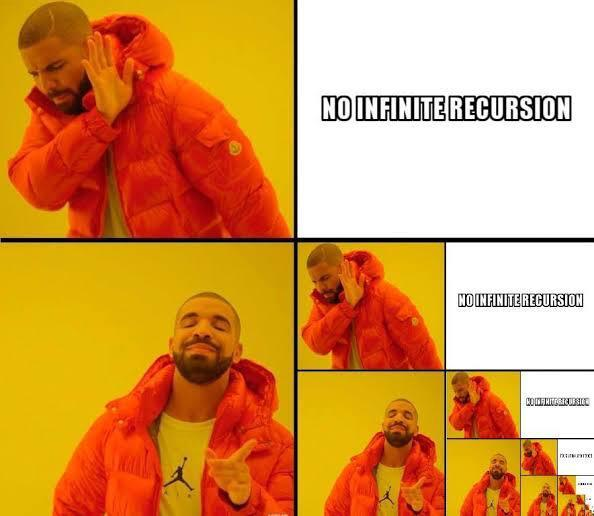Open In App
Related Articles

# Recursion in Python

The term Recursion can be defined as the process of defining something in terms of itself. In simple words, it is a process in which a function calls itself directly or indirectly.• A complicated function can be split down into smaller sub-problems utilizing recursion.
• Sequence creation is simpler through recursion than utilizing any nested iteration.
• Recursive functions render the code look simple and effective.

• A lot of memory and time is taken through recursive calls which makes it expensive for use.
• Recursive functions are challenging to debug.
• The reasoning behind recursion can sometimes be tough to think through.

Syntax:

```def func(): <--
|
| (recursive call)
|
func() ----```

Example 1: A Fibonacci sequence is the integer sequence of 0, 1, 1, 2, 3, 5, 8….

## Python3

 `# Program to print the fibonacci series upto n_terms` `# Recursive function``def` `recursive_fibonacci(n):``  ``if` `n <``=` `1``:``      ``return` `n``  ``else``:``      ``return``(recursive_fibonacci(n``-``1``) ``+` `recursive_fibonacci(n``-``2``))` `n_terms ``=` `10` `# check if the number of terms is valid``if` `n_terms <``=` `0``:``  ``print``(``"Invalid input ! Please input a positive value"``)``else``:``  ``print``(``"Fibonacci series:"``)``for` `i ``in` `range``(n_terms):``    ``print``(recursive_fibonacci(i))`

Output

```Fibonacci series:
0
1
1
2
3
5
8
13
21
34```

Example 2: The factorial of 6 is denoted as 6! = 1*2*3*4*5*6 = 720.

## Python3

 `# Program to print factorial of a number``# recursively.` `# Recursive function``def` `recursive_factorial(n):``  ``if` `n ``=``=` `1``:``      ``return` `n``  ``else``:``      ``return` `n ``*` `recursive_factorial(n``-``1``)` `# user input``num ``=` `6` `# check if the input is valid or not``if` `num < ``0``:``  ``print``(``"Invalid input ! Please enter a positive number."``)``elif` `num ``=``=` `0``:``  ``print``(``"Factorial of number 0 is 1"``)``else``:``  ``print``(``"Factorial of number"``, num, ``"="``, recursive_factorial(num))`

Output

`Factorial of number 6 = 720`

## What is Tail-Recursion?

A unique type of recursion where the last procedure of a function is a recursive call. The recursion may be automated away by performing the request in the current stack frame and returning the output instead of generating a new stack frame. The tail-recursion may be optimized by the compiler which makes it better than non-tail recursive functions.

Is it possible to optimize a program by making use of a tail-recursive function instead of non-tail recursive function?
Considering the function given below in order to calculate the factorial of n, we can observe that the function looks like a tail-recursive at first but it is a non-tail-recursive function. If we observe closely, we can see that the value returned by Recur_facto(n-1) is used in Recur_facto(n), so the call to Recur_facto(n-1) is not the last thing done by Recur_facto(n).

## Python3

 `# Program to calculate factorial of a number``# using a Non-Tail-Recursive function.` `# non-tail recursive function``def` `Recur_facto(n):``  ` `    ``if` `(n ``=``=` `0``):``        ``return` `1``  ` `    ``return` `n ``*` `Recur_facto(n``-``1``)``  ` `# print the result``print``(Recur_facto(``6``))`

Output

`720`

We can write the given function Recur_facto as a tail-recursive function. The idea is to use one more argument and in the second argument, we accommodate the value of the factorial. When n reaches 0, return the final value of the factorial of the desired number.

## Python3

 `# Program to calculate factorial of a number``# using a Tail-Recursive function.` `# A tail recursive function``def` `Recur_facto(n, a ``=` `1``):``  ` `    ``if` `(n ``=``=` `0``):``        ``return` `a``  ` `    ``return` `Recur_facto(n ``-` `1``, n ``*` `a)``  ` `# print the result``print``(Recur_facto(``6``))`

Output

`720`